### Introduction:

As common adage says ‘the money does not bring happiness’ the author decided to prove this statement with the use of statistical inference methods. Happiness consists of many elements, among others job satisfaction is one of the key element playing significant role in human’s life. Therefore the analysis will try to answer the following research question: Is there a relationship between the level of family income and the level of job satisfaction?

This theme seems to be interesting as the answer should help to understand and consider whether families with high income are happier due to higher level of job satisfaction. The answer can also help in choosing the life goals. Most people seem to have strong requirements in terms of performing profession as well as salary expectations. More money means better and more interesting job? More money always make us happier?

### Data:

Data Collection

Used data come from General Social Survey, period 2000 - 2012. This is a survey performed in face to face method by interviewers in sampled households to collect data on demographic characteristics and attitudes of residents of the United States.The survey has been executed by the National Opinion Research Center at the University of Chicago of adults (18+).

The units of observation are adults in randomly selected households in USA.

Variables

Family Income - numerical continous variable in USD

Job Satissfaction - states for the level of satissfaction from performed job, categorical ordinal variable with 4 levels:

1. Very satisfied
2. Mod. Satisfied
3. A little dissatisfied
4. Very Dissatified

Study

This is an observational study, as researchers simply go and collect data based on what is seen and heard and then any inference is not being made. Variables are simply observed and data is collected through an observation. Researchers do not interfere with the data. In this study there is no control and treatment groups like in an experiment.

Scope of inference - generalizability and causality

As the units are US adults data can be generalised to the entire population as it is an observational study, less than 10% population included, random sampling. A few examples of biases shall be listed: Convenience bias as not all residents were contacted, non response bias as not all responders responded to these specific quesitons.

The casual link can not be establised between variables as this is an observational study and no confounding variables have been introduced.

### Exploratory data analysis:

In order to investigate the data from the entire GSS database a subset data has been performed. Only variables listed above have been used, omitting any non response items.

Variables are being analyzed separately.

Summary and Visualisation

newdata <- subset(gss, year >= 2000, select=c(coninc, satjob))
final <- newdata[complete.cases(newdata),]
summary(final$coninc) ## Min. 1st Qu. Median Mean 3rd Qu. Max. ## 383 21960 42130 53140 69750 178700 boxplot.default(final$coninc,col = "grey", ylab = "Family Income - USD")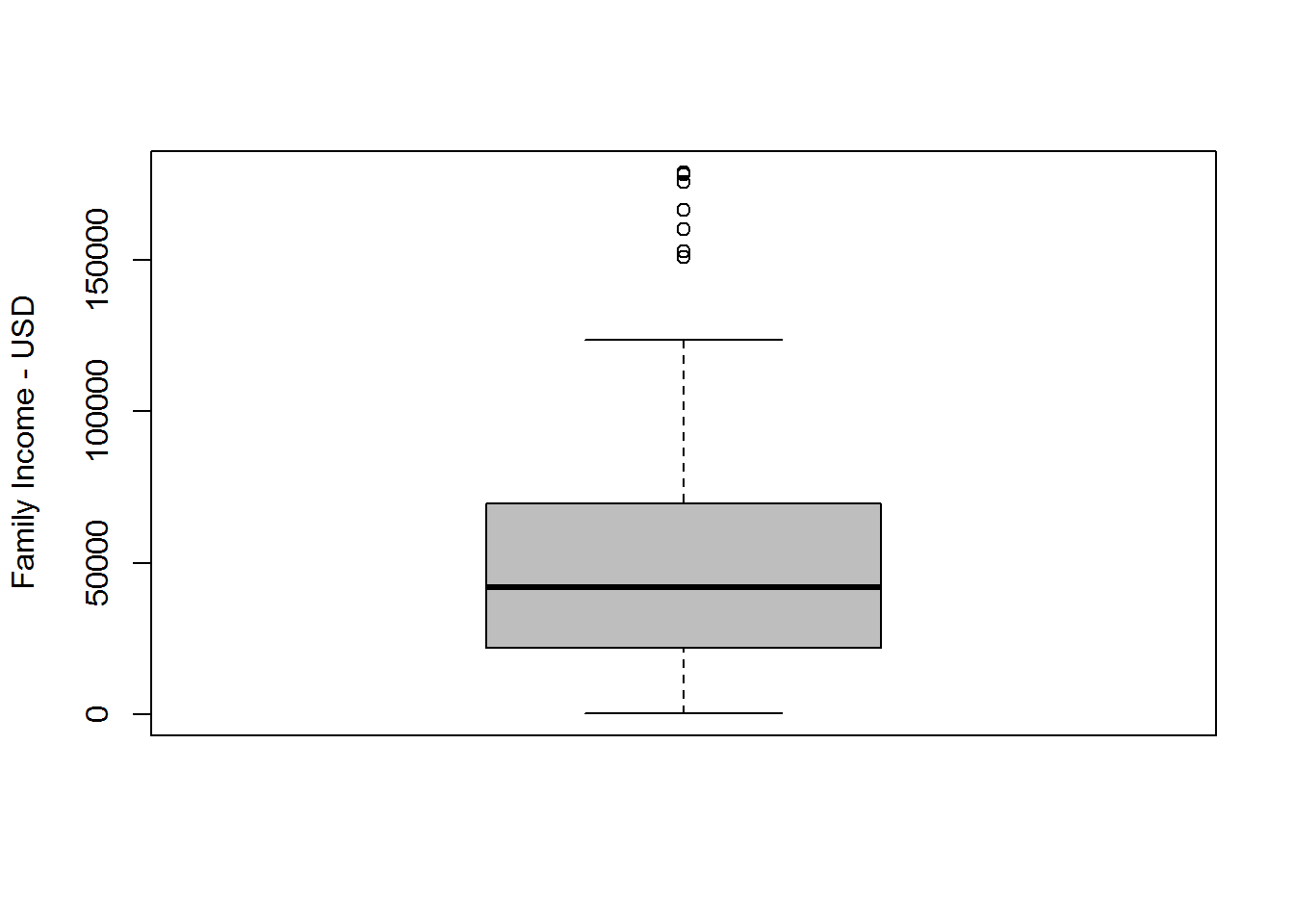hist(final$coninc, col="grey",main="Family Income Distribution 2000-2012",xlab="Family Income")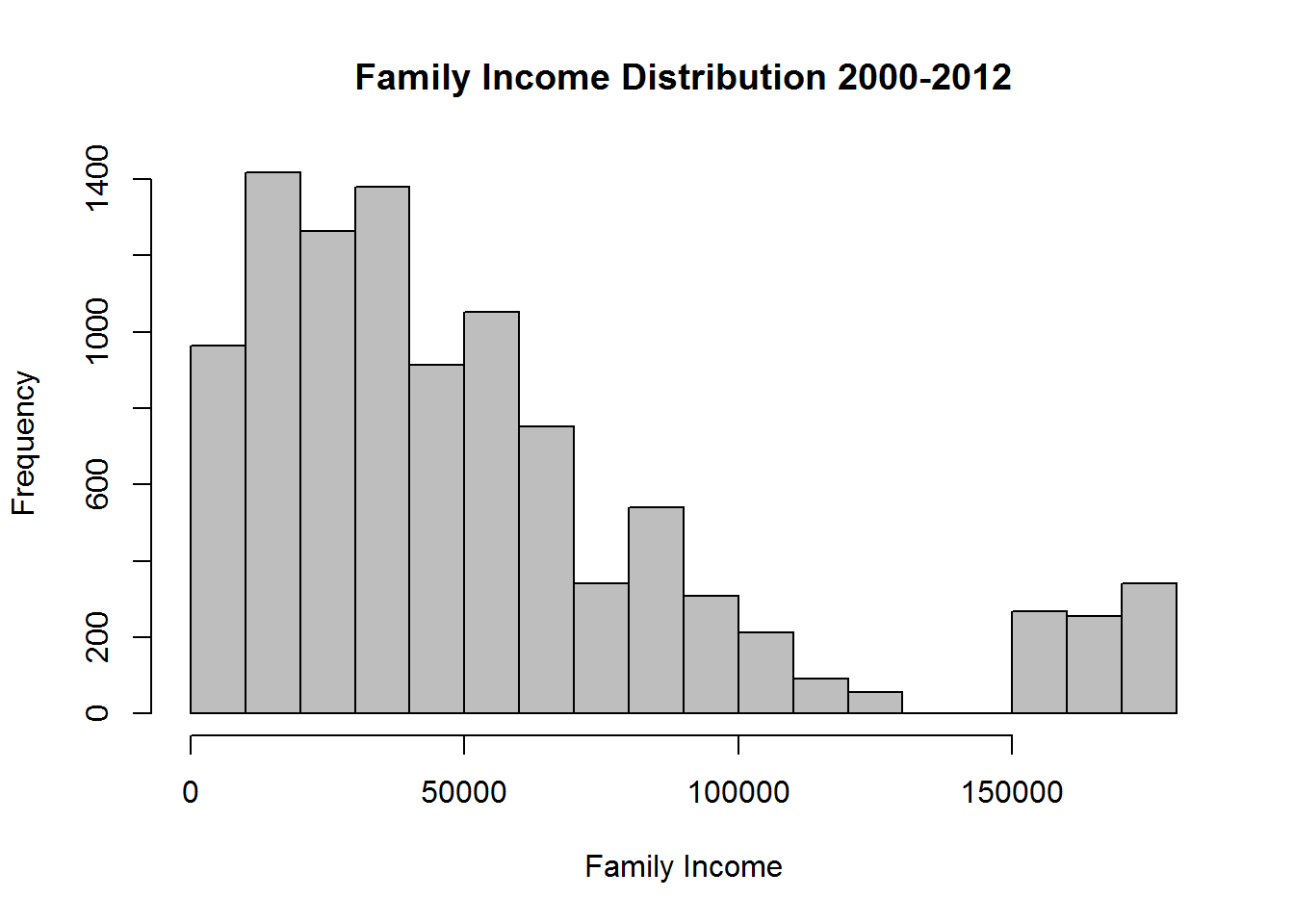hist(final$coninc,probability = TRUE, col="grey",main="Family Income Density 2000-2012",xlab="Family Income")
lines(density(final$coninc), col="blue", lwd=2) lines(density(final$coninc, adjust=10), lty="dotted", col="darkgreen", lwd=2)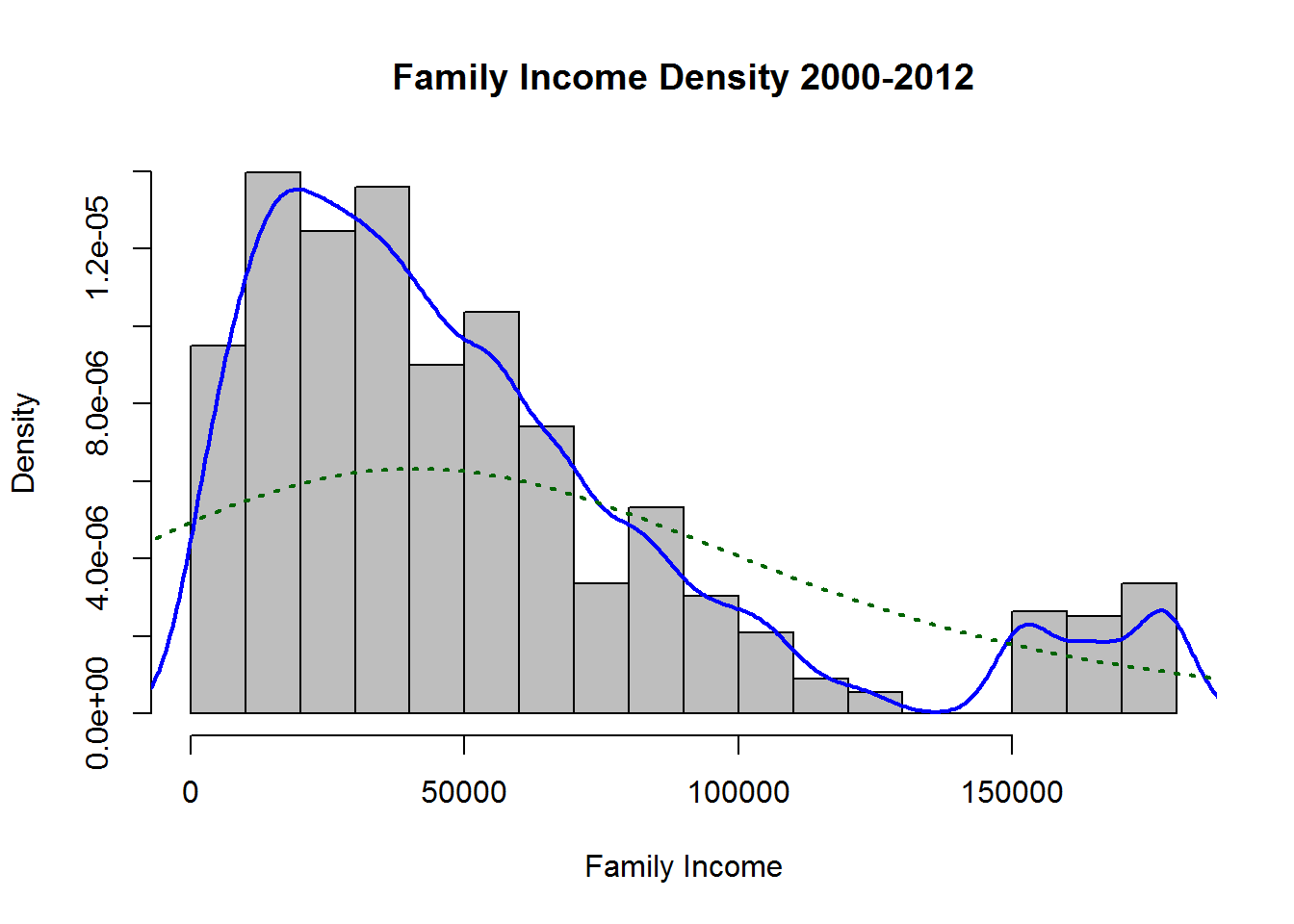As shown on basic summaries the histogram of family income is right skewed and boxplot reveals some outliers. Income range is significantly wide therefore in the measured sample most working classes are included.

The summary of second variable is as follows:

table(final$satjob) ## ## Very Satisfied Mod. Satisfied A Little Dissat Very Dissatisfied ## 4947 3891 930 386 barplot(table(final$satjob),main = "Job Satissfaction - 2000-2012",ylab = "Frequency")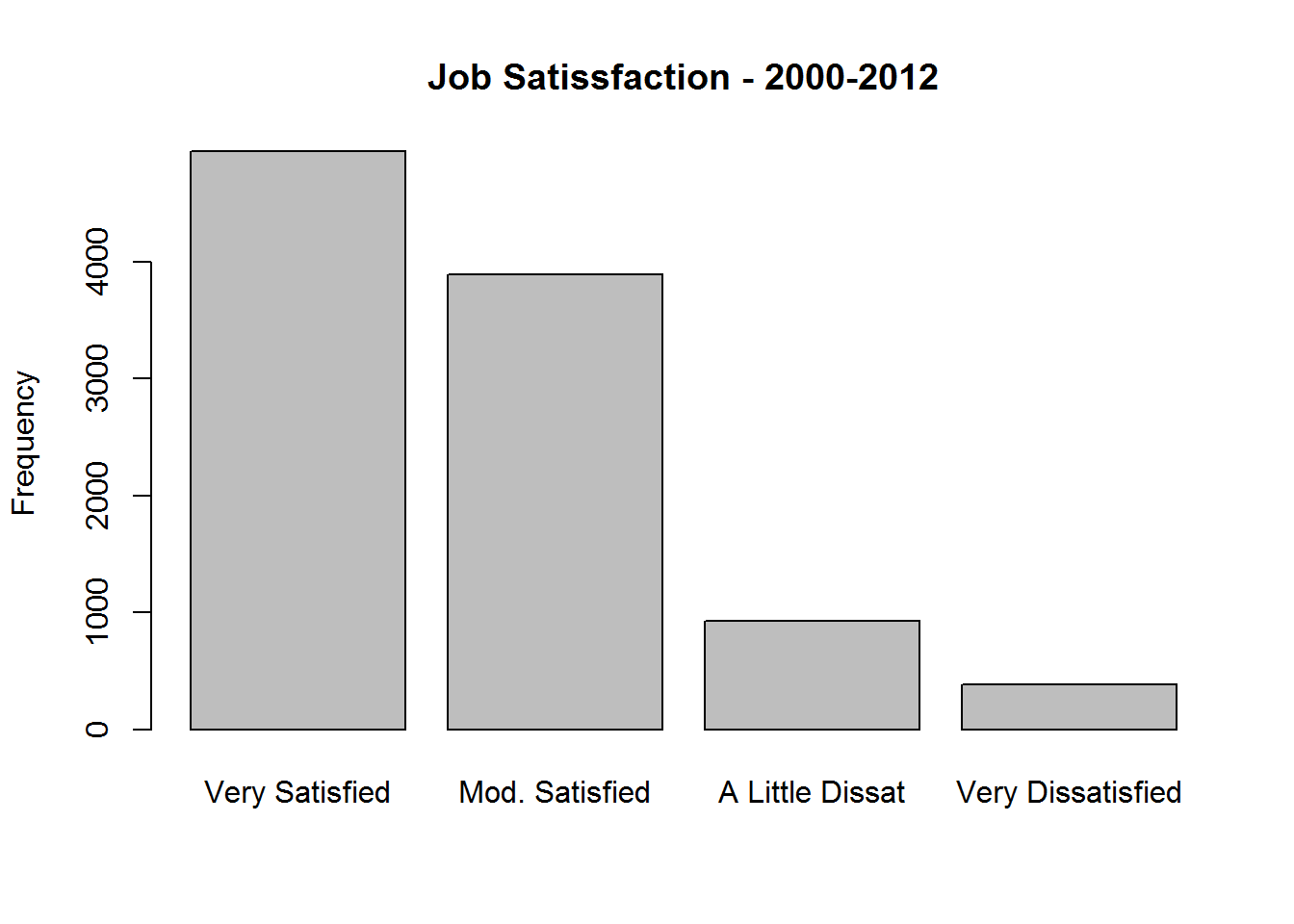pie(prop.table(table(final$satjob)))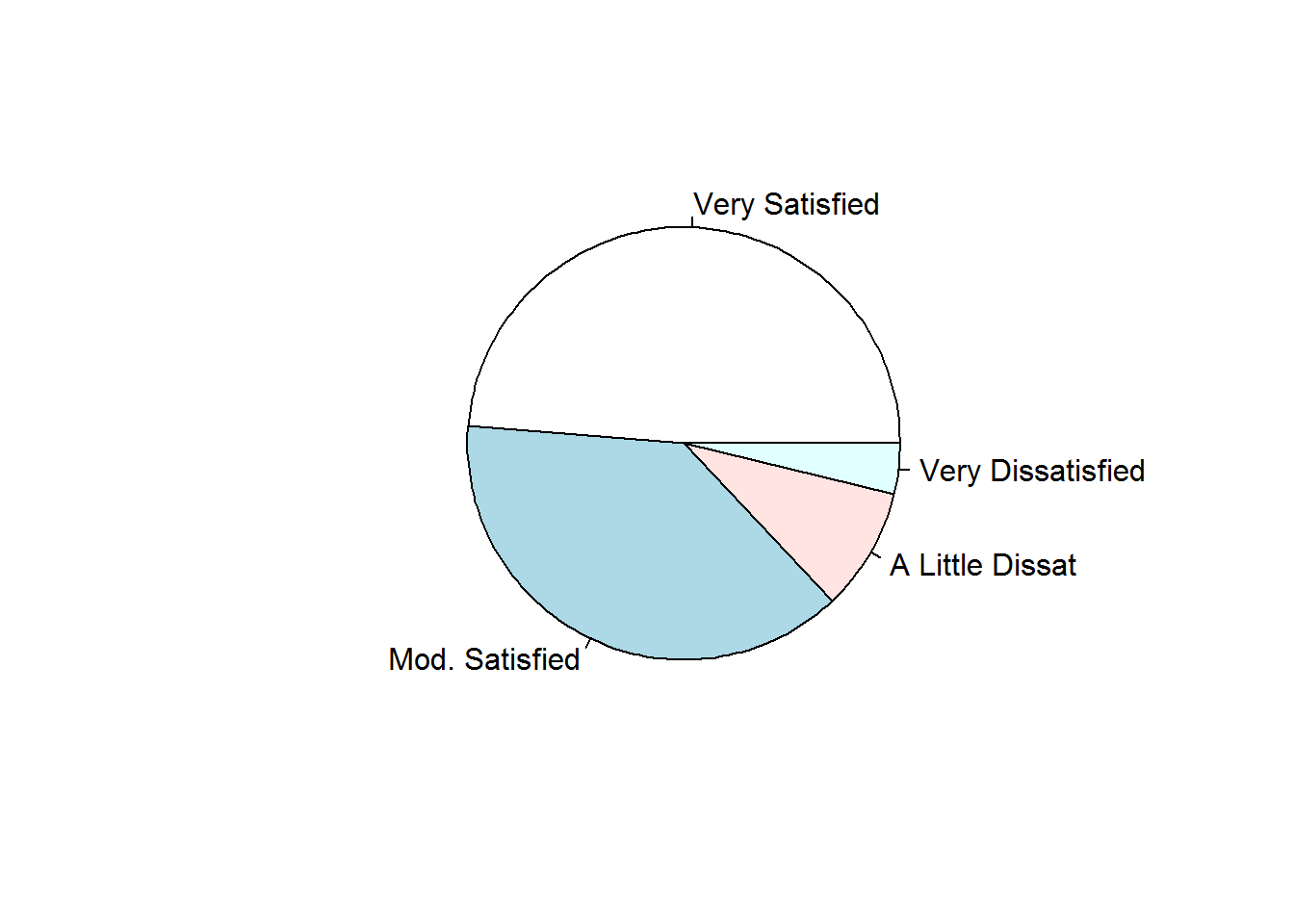Presented charts show that almost 50% of all respondednts are “very Satisfied” with the current job. Still it is not clear what is the income level in this group. Next few charts will clarify briefly the answer to this question. by(final$coninc,final$satjob,summary) ## final$satjob: Very Satisfied
##    Min. 1st Qu.  Median    Mean 3rd Qu.    Max.
##     383   24900   45680   58010   76600  178700
## --------------------------------------------------------
## final$satjob: Mod. Satisfied ## Min. 1st Qu. Median Mean 3rd Qu. Max. ## 383 21060 39700 51240 68520 178700 ## -------------------------------------------------------- ## final$satjob: A Little Dissat
##    Min. 1st Qu.  Median    Mean 3rd Qu.    Max.
##     383   15570   29420   42770   56840  178700
## --------------------------------------------------------
## final$satjob: Very Dissatisfied ## Min. 1st Qu. Median Mean 3rd Qu. Max. ## 383 11750 24260 34740 45680 178300 myfactor<-factor(final$satjob, levels=c("Very Dissatisfied", "A Little Dissat", "Mod. Satisfied","Very Satisfied"))
boxplot(final$coninc~myfactor,col = "grey", ylab = "Family Income - USD")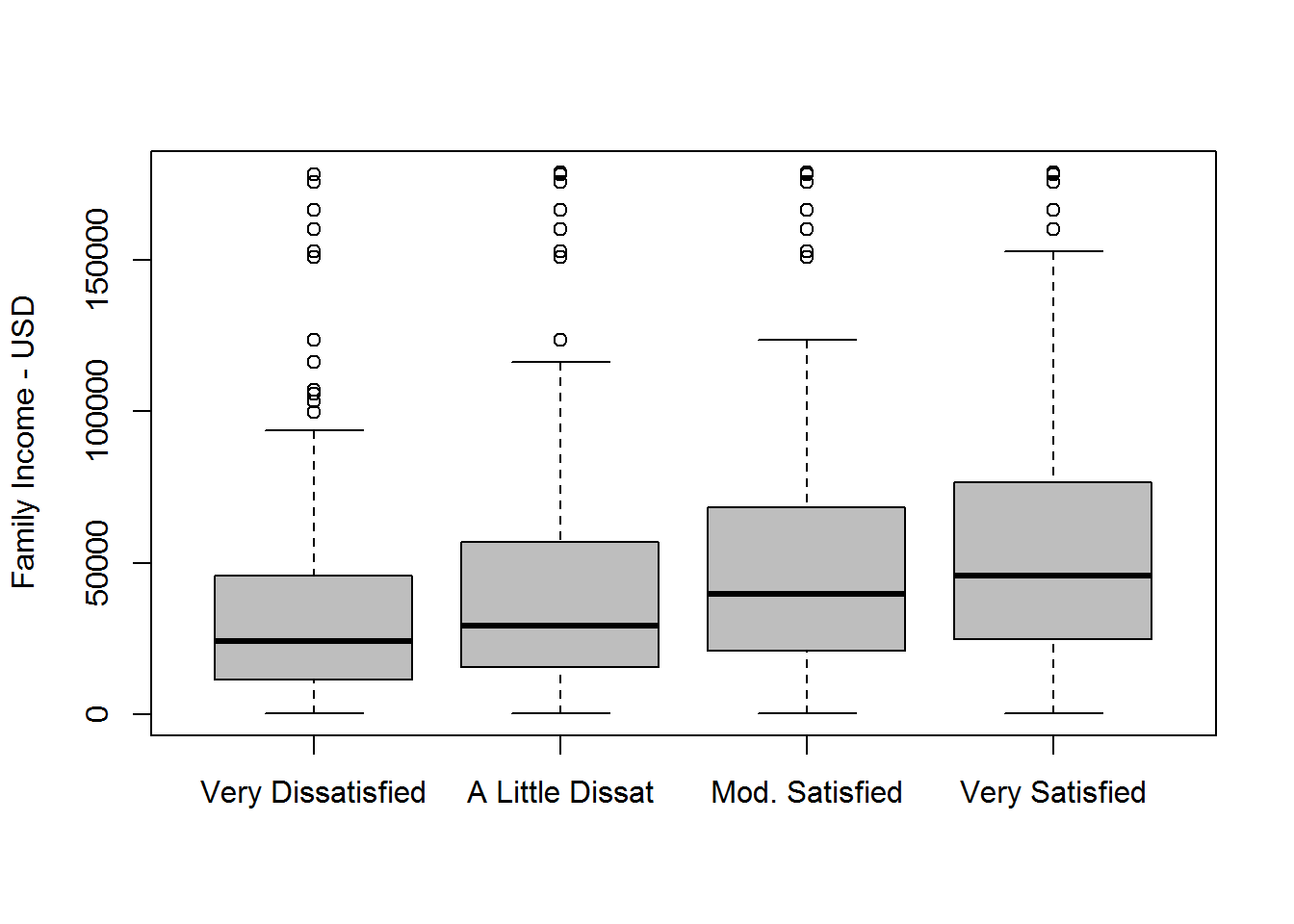The charts seem to reveal positive association between income level and satisfaction with relevant outliers. Nevertheless graphs does not provide significant level of confidence in order to make the final decision. Therefore, next the statistical hypothesis testing will be performed. The charts provide only some taste of the final outcome. ### Inference: Method As in the research continous and discrete variable appear with more than two levels, ANOVA (analysis of variance) should be used as the most adequate hypothesis test, where differences of sample means will be compared. State hypothesis H0 : Job satissfaction and level of family income are independent. Job satisfaction does not vary by the level of income in all groups. HA : Job satissfaction and level of family income are dependent. Job satisfaction does vary by the level of income. At least one pair of means vary from each other. Checking conditions 1. Independence • sample observations within groups and between groups should be independent of each other. This condition is met as variables come from random sampling and chosen sample represents less than 10% of the entire population • random sample - our actual data come from random sampling 1. Distribution of response variable within groups should be approximately normal. This condition can be checked with the use of Q-Q charts. verys <- subset(final, satjob =="Very Dissatisfied") alit <- subset(final, satjob =="A Little Dissat") modsat <- subset(final, satjob =="Mod. Satisfied") veryds <- subset(final, satjob =="Very Satisfied") par(mfrow=c(2,2)) qqnorm(verys$coninc, main = "Very Dissatisfied")
qqline(verys$coninc, col="blue") qqnorm(alit$coninc, main = 'A little Dissat')
qqline(alit$coninc, col="blue") qqnorm(modsat$coninc, main = 'Mod. Satisfied')
qqline(modsat$coninc, col="blue") qqnorm(veryds$coninc, main = 'Very Satisfied')
qqline(veryds$coninc, col="blue")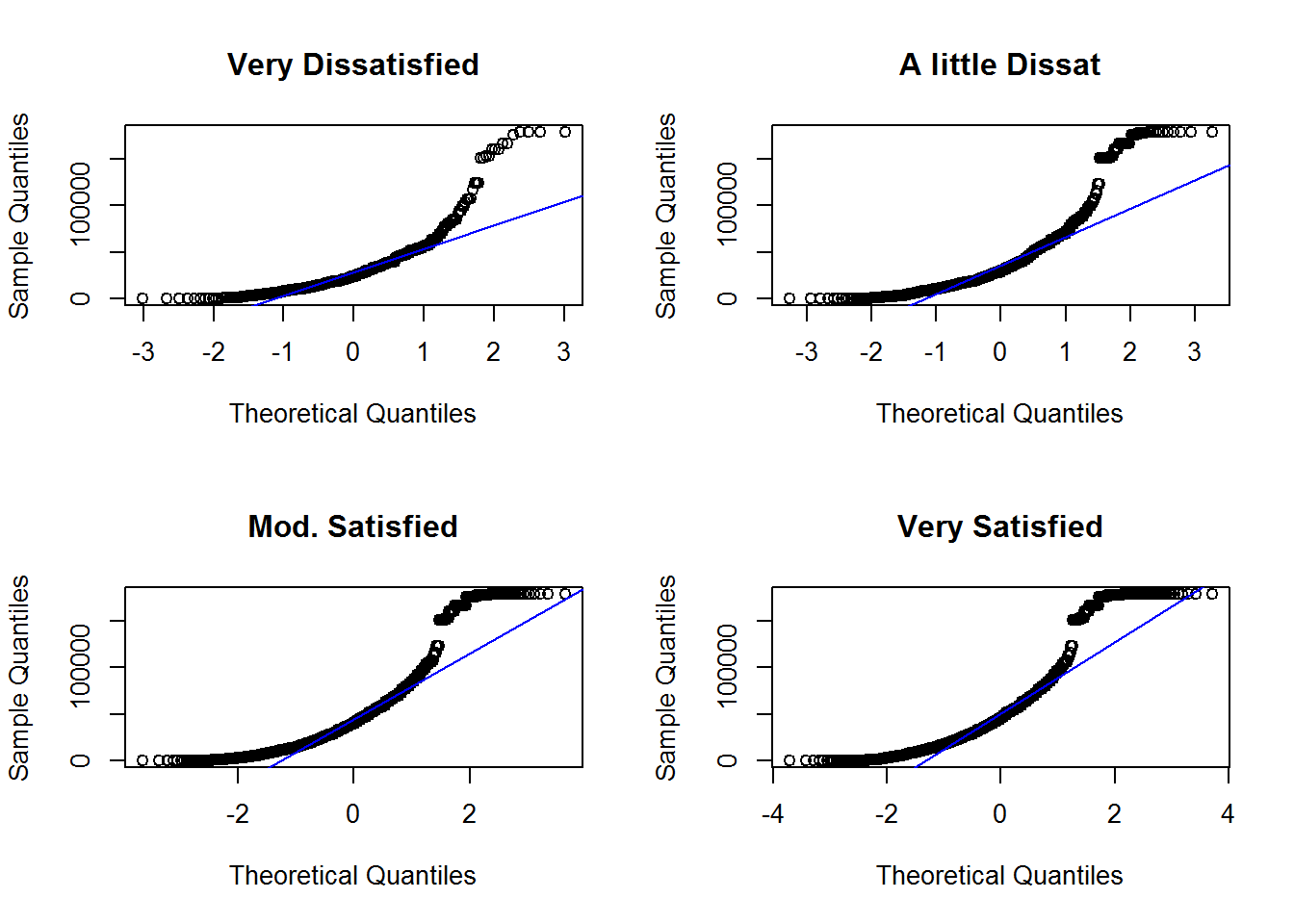As observed data within each group are not ideally normally distributed. Expected line should be straight as in normal probability plot. However the sample size is relatively large, therefore the distribution can be accepted in this research. 1. The last condition relates to variability within each group, which is supposed to be roughly equal. by(final$coninc, final$satjob, sd) ## final$satjob: Very Satisfied
##  45578.68
## --------------------------------------------------------
## final$satjob: Mod. Satisfied ##  41445.46 ## -------------------------------------------------------- ## final$satjob: A Little Dissat
##  40688.84
## --------------------------------------------------------
## final$satjob: Very Dissatisfied ##  35139.27 As observed, standard deviation is relatively equal in each group. ANOVA (Analysis of Variance) is performed to measure p-value and F statistic for this test. If p value is lower than 0.05 we will reject Ho. source("http://bit.ly/dasi_inference") inference(y=final$coninc, x=final$satjob, est="mean", type="ht", null=0, alternative="greater",method="theoretical") ## Warning: package 'openintro' was built under R version 3.2.3 ## Warning: package 'BHH2' was built under R version 3.2.3 ## Response variable: numerical, Explanatory variable: categorical ## Warning: Ignoring null value since it's undefined for ANOVA. ## ANOVA ## Summary statistics: ## n_Very Satisfied = 4947, mean_Very Satisfied = 58011.4, sd_Very Satisfied = 45578.68 ## n_Mod. Satisfied = 3891, mean_Mod. Satisfied = 51241, sd_Mod. Satisfied = 41445.46 ## n_A Little Dissat = 930, mean_A Little Dissat = 42770.78, sd_A Little Dissat = 40688.84 ## n_Very Dissatisfied = 386, mean_Very Dissatisfied = 34742.39, sd_Very Dissatisfied = 35139.27 ## H_0: All means are equal. ## H_A: At least one mean is different. ## Analysis of Variance Table ## ## Response: y ## Df Sum Sq Mean Sq F value Pr(>F) ## x 3 3.6207e+11 1.2069e+11 64.575 < 2.2e-16 ## Residuals 10150 1.8970e+13 1.8690e+09 ## ## Pairwise tests: t tests with pooled SD ## Very Satisfied Mod. Satisfied A Little Dissat ## Mod. Satisfied 0 NA NA ## A Little Dissat 0 0 NA ## Very Dissatisfied 0 0 0.0022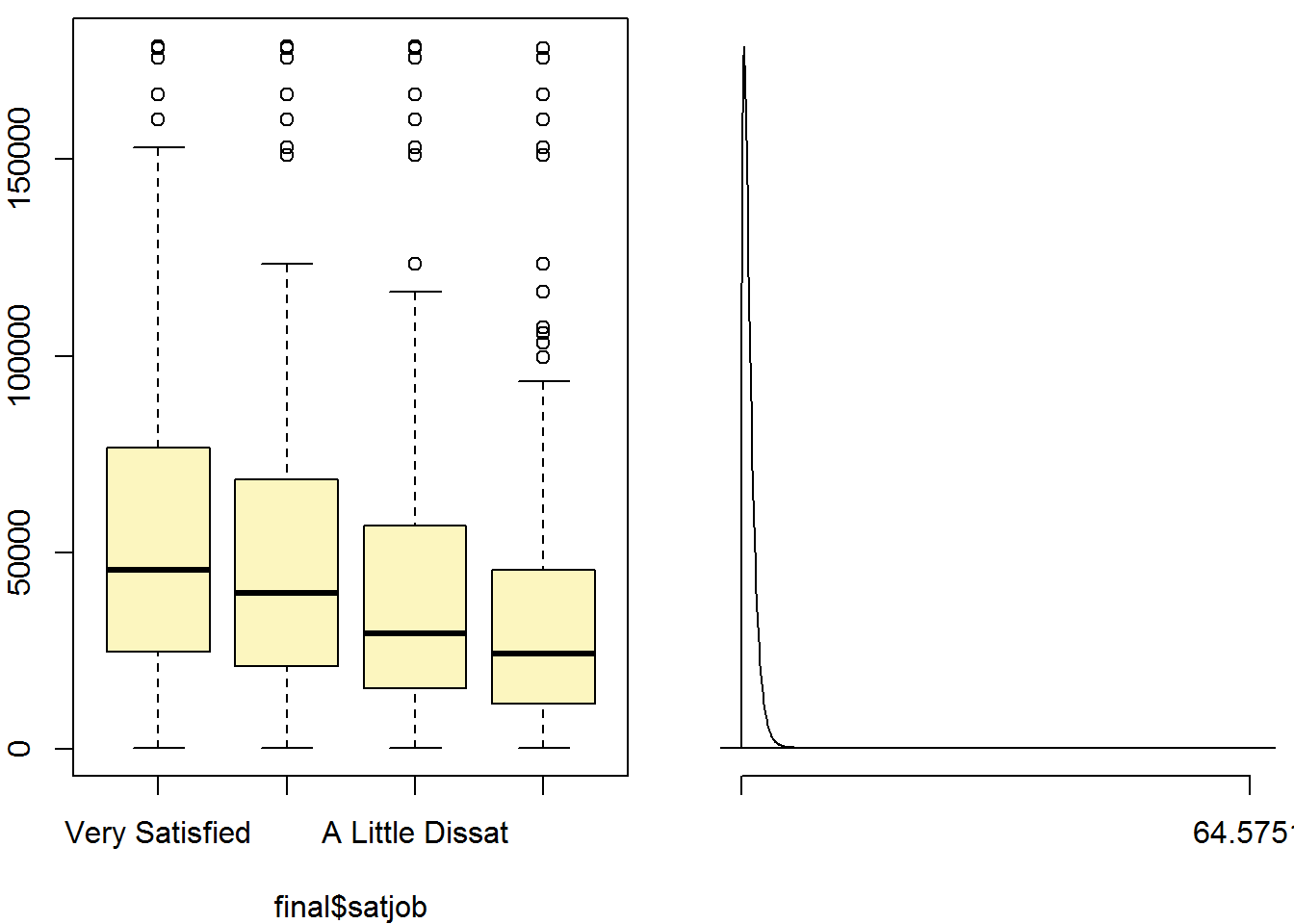As per ANOVA definition total variability consists of two parts: 1. between groups - attributed to groups 2. within groups - attributed to other, unknown factors To reject H0 the p-value needs to be small, which results in large F statistic, which means the variability between groups is larger than the variability within groups. According to the inference the p-value is significantly low, therefore H0 needs to be rejected. The data analysis provide evidence that at least one pair of means in different groups is different from each other. This confidence is very close to 100%. ### Conclusion: Based on hypothesis test performed the only one conclusion should be stated. There are differences between groups, which to some extent proves the statement the money brings happiness. In other words the more we earn the happier we are, the more satisfied we are with performing profession. We are not able to state causation as the research is not en experiment, but an observational study, nevertheless it seems to be correlation between the level of income and job satisfaction. However it is not proved yet that job satisfaction is a key component of happiness. Therefore an addtional study should be performed in which respondents would list all “happiness components” and then regression model would prove which Xs play key role in building happiness feeling. ### Appendix: head(data.frame(gss$coninc,gss\$satjob),n=40L)
##    gss.coninc      gss.satjob
## 1       25926 A Little Dissat
## 2       33333            <NA>
## 3       33333  Mod. Satisfied
## 4       41667  Very Satisfied
## 5       69444            <NA>
## 6       60185  Mod. Satisfied
## 7       50926  Very Satisfied
## 8       18519 A Little Dissat
## 9        3704  Mod. Satisfied
## 10      25926  Mod. Satisfied
## 11      18519            <NA>
## 12      18519  Very Satisfied
## 13      18519  Very Satisfied
## 14      18519  Mod. Satisfied
## 15      25926  Very Satisfied
## 16      18519  Mod. Satisfied
## 17      33333            <NA>
## 18      25926            <NA>
## 19      60185  Very Satisfied
## 20      69444            <NA>
## 21      50926  Very Satisfied
## 22      83333  Very Satisfied
## 23      18519  Mod. Satisfied
## 24      25926            <NA>
## 25      41667            <NA>
## 26      41667  Mod. Satisfied
## 27      41667  Mod. Satisfied
## 28      41667 A Little Dissat
## 29         NA  Mod. Satisfied
## 30      41667  Very Satisfied
## 31      33333  Mod. Satisfied
## 32      33333  Very Satisfied
## 33      41667            <NA>
## 34       3704            <NA>
## 35      18519  Mod. Satisfied
## 36      41667  Mod. Satisfied
## 37      69444            <NA>
## 38      41667            <NA>
## 39      25926  Mod. Satisfied
## 40      18519  Mod. Satisfied